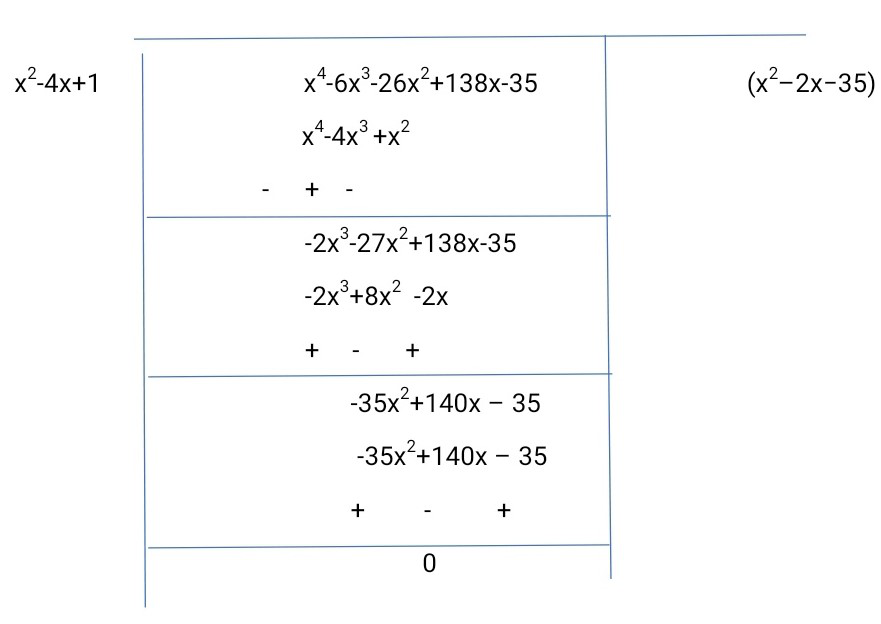# Class 10 NCERT Solutions – Chapter 2 Polynomials – Exercise 2.4

### (i) 2x3 + x2 – 5x + 2; 1/2, 1, -2

Solution:

p(x) = 2x3+x2-5x+2

p(1/2) = 2(1/2)3+(1/2)2-5(1/2)+2

= (1/4)+(1/4)-(5/2)+2

= 0

p(1) = 2(1)3+(1)2-5(1)+2 = 0

p(-2) = 2(-2)3+(-2)2-5(-2)+2 = 0

Therefore, 1/2, 1, -2 are the zeroes of 2x3+x2-5x+2.

Now, comparing the given polynomial with general expression

ax3+bx2+cx+d = 2x3+x2-5x+2

a=2, b=1, c= -5 and d = 2

α, β, γ are the zeroes of the cubic polynomial ax3+bx2+cx+d

α +β+γ = –b/a

αβ+βγ+γα = c/a

α βγ = – d/a.

α+β+γ = ½+1+(-2)

= -1/2 = –b/a

αβ+βγ+γα = (1/2×1)+(1 ×-2)+(-2×1/2)

= -5/2 = c/a

α β γ = ½×1×(-2)

= -2/2 = -d/a

Hence, the relationship between the zeroes and the coefficients are satisfied.

### (ii) x3 – 4x2 + 5x – 2 ;2, 1, 1

Solution:

p(x) = x3-4x2+5x-2

Zeroes are 2,1,1.

p(2)= 23-4(2)2+5(2)-2

= 0

p(1) = 13-(4)(12 )+(5)(1)-2 = 0

Therefore, proved, 2, 1, 1 are the zeroes of x3-4x2+5x-2

On comparing the given polynomial with general expression

ax3+bx2+cx+d = x3-4x2+5x-2

a = 1, b = -4, c = 5 and d = -2

Therefore,

α + β + γ = –b/a

= 2+1+1

= 4

–b/a = -(-4)/1

αβ + βγ + γα = c/a

= 2×1+1×1+1×2

= 5

c/a = 5/1

α β γ = – d/a.

= 2×1×1

= 2

-d/a = -(-2)/1

Hence, the relationship between the zeroes and the coefficients is satisfied.

### Question 2. Find a cubic polynomial with the sum, sum of the product of its zeroes taken two at a time, and the product of its zeroes as 2, –7, –14 respectively.

Solution:

Let us consider the cubic polynomial as ax3+bx2+cx+d and zeroes of the polynomials be α, β, γ.

α+β+γ = -b/a = 2/1

αβ +βγ+γα = c/a = -7/1

α βγ = -d/a = -14/1

On comparing

a = 1, b = -2, c = -7, d = 14

Therefore, the cubic polynomial is x3-2x2-7x+14

### Question 3. If the zeroes of the polynomial x3 – 3x2 + x + 1are a – b, a, a + b, find a and b.

Solution:

p(x) = x3-3x2+x+1

Zeroes are given as a – b, a, a + b

px3+qx2+rx+s = x3-3x2+x+1

On comparing

p = 1, q = -3, r = 1 and s = 1

Sum of zeroes = a – b + a + a + b

-q/p = 3a

Putting the values q and p.

-(-3)/1 = 3a

a=1

Therefore, zeroes are 1-b, 1, 1+b.

Product of zeroes = 1(1-b)(1+b)

-s/p = 1-b2

-1/1 = 1-b2

b2 = 1+1 = 2

b = √2

Therefore,1-√2, 1,1+√2 are the zeroes of x3-3x2+x+1.

### Question 4. If two zeroes of the polynomial x4-6x3-26x2+138x-35are 2 ±√3,find other zeroes.

Solution:

Degree of polynomial is 4

Therefore, it has four roots

f(x) = x4-6x3-26x2+138x-35

As 2 +√3 and 2-√3 are zeroes of given polynomial f(x).

Therefore, [x−(2+√3)] [x−(2-√3)] = 0

(x−2−√3)(x−2+√3) = 0

Therefore, x2-4x+1 is a factor of polynomial f(x).

Let it be g(x) = x2-4x+1

By dividing f(x) by g(x) we get another factor of f(x)x4-6x3-26x2+138x-35 = (x2-4x+1)(x2 –2x−35)

On factorizing (x2–2x−35) by splitting the middle term

x2–(7−5)x −35 = x2– 7x+5x-35

=x(x −7)+5(x−7)

(x+5)(x−7) = 0

x= −5 and x = 7.

Therefore, all four zeroes of given polynomial equation are: 2+√3, 2-√3, −5 and 7.

Whether you're preparing for your first job interview or aiming to upskill in this ever-evolving tech landscape, GeeksforGeeks Courses are your key to success. We provide top-quality content at affordable prices, all geared towards accelerating your growth in a time-bound manner. Join the millions we've already empowered, and we're here to do the same for you. Don't miss out - check it out now!

Previous
Next